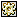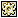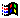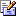Contents Articles Behaviors Books Director News Director Web Sites FAQ Games Mailing Lists News Groups Project Examples Reviews Software Tools Useful Web Sites Utilities Xtras

 Don't miss these#Semi-Random Bezier Animatior

 Compatibilities:This item has not yet been rated

Author: DarrelPlant

Semi-Random Bezier Animatior

 property bezchan, bezpos, bezperiod, bezfinish, beztime property p0, p1, p2, p3, da, db, dc on getPropertyDescriptionList      set p_list = [#bezperiod: [ #comment:   "Animation Period:",  #format:   #integer, #default:   60 ], #p3: [ #comment:   "Destination:", #format:   #point, #default:    point (300, 200) ]]   return p_list      end on beginSprite me   set bezchan = the spriteNum of me   set bezpos = the loc of sprite bezchan   set p0 = bezpos   planmove me, p3 end new on randomsign   return random (3) - 2 end randomsign on planmove me, where   set planstage = 0   set p0 = the loc of sprite bezchan   set vector = p0 - p3   set deviation = (veclength (vector) / 2.5) + 1   set p1 = p0 + (vector / 3) + randomsign () * ¬                 point(random (deviation),random(deviation))   set p2 = p3 - (vector / 3) + randomsign () * ¬                 point(random (deviation),random(deviation))   set dc = 3 * (p1 - p0)   set db = 3 * (p2 - p1) - dc   set da = p3 - p0 - dc - db   set beztime = the timer   set bezfinish = beztime + abs (bezperiod) end planmove on veclength aVector   set anH = the locH of aVector   set aV = the locV of aVector   return sqrt (anH * anH + aV * aV) end vecLength on planimate me   set atime = float (the timer - beztime)   if atime <= bezperiod then     set t1 = atime / bezperiod     set t2 = t1 * t1     set t3 = t2 * t1     set bezpos = da * t3 + db * t2 + dc * t1 + p0     set bezpos = point (the loch of bezpos, the locv of bezpos)     set the loc of sprite bezchan = bezpos   else     if bezpos <> p3 then       set bezpos = p3       set the loc of sprite bezchan = bezpos     end if   end if end planimate on exitframe me   planimate me end stepframe on getBehaviorDescription me   set line1 = "Creates a semi-random Bezier curve animation path between the sprite"s original position "   set line2 = "and a point determined in the property dialog box. The period of the animation is "   set line3 = "user-definable (measured in ticks)." & RETURN   set line4 = "The variation of the Bezier curve is dependent on the distance moved. For more info on "   set line5 = "Bezier animation techniques in Director, see http://www.moshplant.com/direct-or/bezier/"   return line1 & line2 & line3 & line4 & line5 end

 Contact MMI 36 South Court Sq Suite 300 Newnan, GA 30263 USASend e-mail